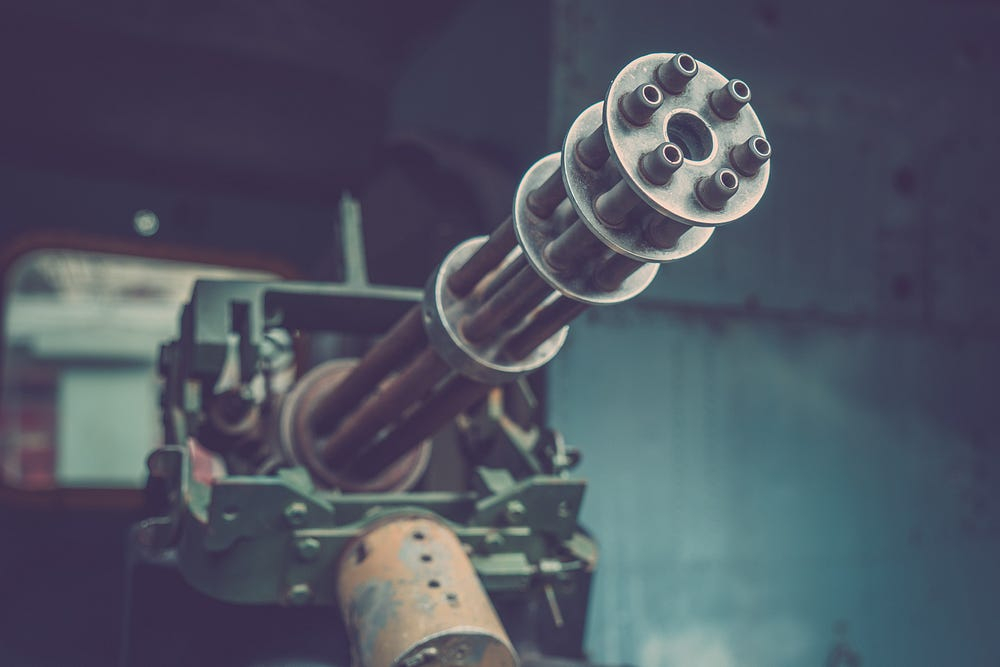# Destroy Image Classification by Ensemble of Pre-trained models

Original article was published on Deep Learning on Medium# Requirements

If you want to code along you will require Tensorflow and OpenCV. You can also use Google Colab like me where all the required packages for our task will be pre-installed and it also offers free GPU.

The dataset chosen to be annihilated is the classic cats vs dogs one. As it is a small dataset we’ll load it completely in the memory so that it trains faster.

`import tensorflow as tfimport osimport numpy as npimport matplotlib.pyplot as pltimport reimport randomimport cv2_URL = 'https://storage.googleapis.com/mledu-datasets/cats_and_dogs_filtered.zip'path_to_zip = tf.keras.utils.get_file('cats_and_dogs.zip', origin=_URL, extract=True)PATH = os.path.join(os.path.dirname(path_to_zip), 'cats_and_dogs_filtered')train_dir = os.path.join(PATH, 'train')validation_dir = os.path.join(PATH, 'validation')train_cats_dir = os.path.join(train_dir, 'cats')train_dogs_dir = os.path.join(train_dir, 'dogs')validation_cats_dir = os.path.join(validation_dir, 'cats')validation_dogs_dir = os.path.join(validation_dir, 'dogs')cats_tr = os.listdir(train_cats_dir)dogs_tr = os.listdir(train_dogs_dir)cats_val = os.listdir(validation_cats_dir)dogs_val = os.listdir(validation_dogs_dir)cats_tr = [os.path.join(train_cats_dir, x) for x in cats_tr]dogs_tr = [os.path.join(train_dogs_dir, x) for x in dogs_tr]cats_val = [os.path.join(validation_cats_dir, x) for x in cats_val]dogs_val = [os.path.join(validation_dogs_dir, x) for x in dogs_val]total_train = cats_tr + dogs_trtotal_val = cats_val + dogs_val`

The paths of all the training and validation (in this case testing) images are stored in total_train and total_val. We will use OpenCV to read the images and store them in NumPy array having dimensions (no of images x image shape x channels). Their corresponding labels will also be stored in a one dimensional NumPy array.

`def data_to_array(total): random.shuffle(total) X = np.zeros((len(total_train), 224, 224, 3)).astype('float') y = [] for i, img_path in enumerate(total): img = cv2.imread(img_path) img = cv2.resize(img, (224, 224)) X[i] = img if len(re.findall('dog', img_path)) == 3: y.append(0) else: y.append(1) y = np.array(y) return X, yX_train, y_train = data_to_array(total_train)X_test, y_test = data_to_array(total_val)`

# Creating the Ensemble Model

## Training Individual Models and Saving them

Our first task would be to create all the individual models. I will be creating three different models using MobileNetV2, InceptionV3, and Xception. Creating a model using a pre-trained network is very easy in Tensorflow. We need to load the weights, decide whether to freeze or unfreeze the loaded weights, and finally add Dense layers to make the output how we want. The basic structure I will be using for my models:

`def create_model(base_model): base_model.trainable = True global_average_layer = tf.keras.layers.GlobalAveragePooling2D()(base_model.output) prediction_layer = tf.keras.layers.Dense(1, activation='sigmoid')(global_average_layer) model = tf.keras.models.Model(inputs=base_model.input, outputs=prediction_layer) model.compile(optimizer=tf.keras.optimizers.Adam(lr=0.0001), loss=tf.keras.losses.BinaryCrossentropy(from_logits=True), metrics=["accuracy"]) return model`

After creating our models we need to fit them on our training data for some epochs.

`batch_size = 32epochs = 20def fit_model(model): history = model.fit(X_train, y_train, batch_size=batch_size, steps_per_epoch=len(total_train)//batch_size,  epochs=epochs,  validation_data=(X_test, y_test),  validation_steps=len(total_val)//batch_size) return historyIMG_SHAPE = (224, 224, 3)base_model1 = tf.keras.applications.MobileNetV2(input_shape=IMG_SHAPE, include_top=False, weights="imagenet")base_model2 = tf.keras.applications.InceptionV3(input_shape=IMG_SHAPE, include_top=False, weights="imagenet")base_model3 = tf.keras.applications.Xception(input_shape=IMG_SHAPE, include_top=False, weights="imagenet")model1 = create_model(base_model1)model2 = create_model(base_model2)model3 = create_model(base_model3)history1 = fit_model(model1)model1.save('models/model1.h5')history2 = fit_model(model2)model2.save('models/model2.h5')history3 = fit_model(model3)model3.save('models/model3.h5')`

Let us see how our models performed on there own.

The results are not at all bad but we will still improve them.

## Load the Model and Freeze its Layers

Our next step is to load the models we have just created above and freeze their layers so that their weights are not altered when we fit our ensemble model on them.

`def load_all_models(): all_models = [] model_names = ['model1.h5', 'model2.h5', 'model3.h5'] for model_name in model_names: filename = os.path.join('models', model_name) model = tf.keras.models.load_model(filename) all_models.append(model) print('loaded:', filename) return all_modelsmodels = load_all_models()for i, model in enumerate(models): for layer in model.layers: layer.trainable = False`

## Concatenate their outputs and add Dense Layers

Take the outputs of all the models and put them in a concatenation layer. Then add a Dense layer with some units followed by a Dense layer with a single output and an activation equal to “sigmoid” as our task is a binary classification. This can be thought of as an ANN where the predictions of all the models are taken as inputs and an output is provided.

`ensemble_visible = [model.input for model in models]ensemble_outputs = [model.output for model in models]merge = tf.keras.layers.concatenate(ensemble_outputs)merge = tf.keras.layers.Dense(10, activation='relu')(merge)output = tf.keras.layers.Dense(1, activation='sigmoid')(merge)model = tf.keras.models.Model(inputs=ensemble_visible, outputs=output)`

## Compile and Train the Ensemble Model

I used the classic ‘Adam’ optimizer with a little high learning rate of 10x-3 to compile the model.

`model.compile(optimizer=tf.keras.optimizers.Adam(lr=0.001), loss=tf.keras.losses.BinaryCrossentropy(from_logits=True), metrics=["accuracy"])`

Let’s see how our model looks now.

Can we train this normally by just passing the dataset like how we trained our individual models? No! Inputs are required at three places while only one output is generated. So we will need to configure our X values like that.

`X_train = [X_train for _ in range(len(model.input))]X_test = [X_test for _ in range(len(model.input))]`

Now we can fit the model as we had done previously.

`history = model.fit(X, y_train, batch_size=batch_size, steps_per_epoch=len(total_train) // batch_size, epochs=epochs,  validation_data=(X_1, y_test), validation_steps=len(total_val) // batch_size)`

# Results

First, let us plot the graphs for our ensemble model.

I have trained it for just 20 epochs but having a look at the loss curves shows that the curve is still going down and the model can be trained for some more epochs. Let’s see what validation accuracies did the models give on their final epochs.

`MobileNetV2 acc: 0.9788306355476379InceptionV3 acc: 0.9778226017951965Xception acc: 0.9788306355476379Ensemble acc: 0.9828628897666931`

The ensemble accuracy is almost a 0.5% increase which is tremendous especially if taken into account that the accuracies before that were 97.8%.